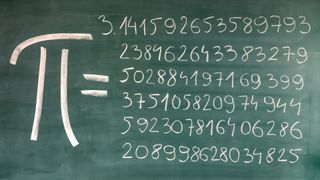# What is pi?Pi is a number that relates a circle's circumference to its diameter. Pi is an irrational number, which means that it is a real number that cannot be expressed by a simple fraction. That's because pi is what mathematicians call an "infinite decimal" — after the decimal point, the digits go on forever and ever.

Students are usually introduced to the number pi as having an approximate value of 3.14 or 3.14159. Though it is an irrational number, some people use rational expressions, such as 22/7 or 333/106, to estimate pi. (These rational expressions are accurate only to a couple of decimal places.)

Mathematicians and math enthusiasts are interested in calculating pi to as many digits as possible. The record for reciting the most digits of pi belongs to Suresh Kumar Sharma of India, who recited pi to 70,030 decimal places in 2015, according to the Pi World Ranking List. Meanwhile, some computer programs have calculated the value of pi to an astounding 62.8 trillion digits, Live Science previously reported. Calculations like these are often unveiled on Pi Day, a pseudo-holiday that occurs every year on March 14 (3/14).

### What are the first 100 digits of pi?

The first 100 digits of pi are:

3.14159 26535 89793 23846 26433 83279 50288 41971 69399 37510 58209 74944 59230 78164 06286 20899 86280 34825 34211 7067

The website piday.org has pi listed to the first 1 million digits.

### Where does pi come from?Some equations on the geometry of circles (Image credit: Barry Barnes via Shutterstock)

By definition, pi is the ratio of the circumference of a circle to its diameter. In other words, pi equals the circumference divided by the diameter (π = c/d). Conversely, the circumference of a circle is equal to pi times the diameter (c = πd). No matter how large or small a circle is, pi will always work out to be the same number. Pi (π) is the 16th letter of the Greek alphabet and is used to represent the widely known mathematical constant.

### Who invented pi?

Pi has been known for nearly 4,000 years and was discovered by the ancient Babylonians. A tablet dating to somewhere between 1900 B.C. and 1680 B.C. found pi to be 3.125, according to the Exploratorium in San Francisco. People in ancient Egypt were making similar discoveries, as evidenced by the Rhind papyrus of 1650 B.C. In this document, the Egyptians calculated the area of a circle by a formula giving pi an approximate value of 3.1605. There is even a biblical verse where it appears pi was approximated, according to a correspondence published in 1999 in the journal Nature:

And he made a molten sea, ten cubits from the one brim to the other: it was round all about, and his height was five cubits: and a line of thirty cubits did compass it about. — I Kings 7:23 (King James Version)

One of the first calculations of pi was carried out by Greek mathematician Archimedes of Syracuse (287 B.C. to 212 B.C.), according to the Exploratorium. Archimedes used the Pythagorean theorem to find the areas of two polygons. Archimedes approximated the area of a circle based on the area of a regular polygon inscribed within the circle and the area of a regular polygon within which the circle was circumscribed. The polygons, as Archimedes mapped them, gave the upper and lower bounds for the area of a circle, and he approximated pi at between 3 1/7 and 3 10/71.An engraving that depicts the last moments of Greek mathematician and inventor Archimedes (c. 287 - 212 BC) as he ponders a mathematical diagram just before his death at the hands of invading Roman soldiers in Syracuse, Sicily, in 212 BC. (Image credit: Stock Montage via Getty Images)

Earlier, Chinese mathematician and astronomer Zu Chongzhi (429 B.C. to 501 B.C.) had calculated pi using a similar method, finding the value to be 355/113. Unfortunately, Zu's book of writing has been lost, so little is known about his work or methods.

British mathematician William Jones was the first to begin using the symbol π to represent pi, in 1706.

### What is pi used for?

In basic mathematics, pi is used to find the area and circumference of a circle. Pi can be used to find an area by multiplying the radius of the circle squared times pi. So, to find the area of a circle with a radius of 3 centimeters, the calculation would be π3^2 = 28.27 cm. Because circles appear commonly in nature and are often used in other mathematical equations, pi is all around us and is used constantly.

Pi has even trickled into the literary world. Pilish is a form of writing English in which the numbers of letters in successive words follow the digits of pi, according to author Mike Keith. Keith used Pilish in his book "Not A Wake." Here is an example from the book: "Now I fall, a tired suburbian in liquid under the trees, Drifting alongside forests simmering red in the twilight over Europe."

"Now" has 3 letters, "I" has 1 letter, "fall" has 4 letters, "a" has 1 letter, and so on.

This article was updated on Oct. 19, 2018, by Live Science senior writer Brandon Specktor. It was updated again on March 3, 2022, by Live Science contributor Adam Mann.

### Bibliography

Exploratorium. (n.d.). A brief history of pi. Retrieved March 3, 2022, from https://www.exploratorium.edu/pi/history-of-pi

Keith, M. (2010). Not a wake. Vinculum Press. Retrieved March 3, 2022, from http://www.cadaeic.net/notawake.htm

Keith, M. (n.d.). Writing in Philish. Retrieved March 3, 2022, from http://www.cadaeic.net/pilish.htm

Mometrix Test Preparation. (n.d.) 1 million digits of pi. Retrieved March 3, 2022, from https://www.piday.org/million

Peil, N. (1999). Biblical answer to cooking up pi. Nature 399(522). https://www.nature.com/articles/21064

Pi World Ranking List. (n.d.). Retrieved March 3, 2022, from https://www.pi-world-ranking-list.com/?page=lists&category=pi

The Nine Planets (2020, October 8). Hilarious pi jokes. https://nineplanets.org/pi-jokes/